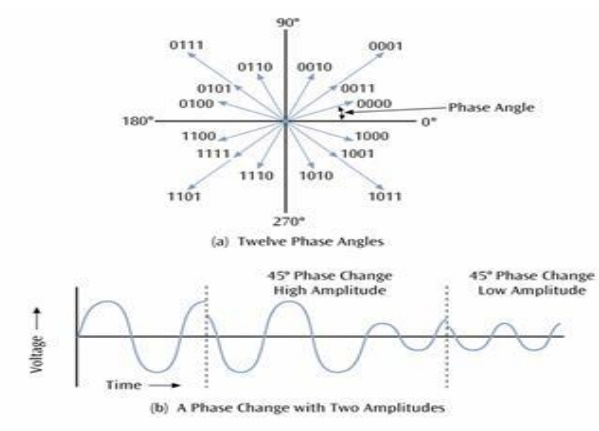Home | | Digital Communication | QAM(Quadrature Amplitude Modulation)

## Chapter: Digital Communication : Digital Modulation Scheme

QAM is a combination of ASK and PSK

• QAM is a combination of ASK and PSK

Two different signals sent simultaneously on the same carrier frequency ie, M=4, 16, 32, 64, 128, 256.

As an example of QAM, 12 different phases are combined with two different amplitudes. Since only 4 phase angles have 2 different amplitudes, there are a total of 16 combinations. With 16 signal combinations, each baud equals 4 bits of information (2 ^ 4 = 16). Combine ASK and PSK such that each signal corresponds to multiple bits. More phases than amplitudes. Minimum bandwidth requirement of QAM is same as ASK or PSK.Study Material, Lecturing Notes, Assignment, Reference, Wiki description explanation, brief detail
Digital Communication : Digital Modulation Scheme : QAM(Quadrature Amplitude Modulation) |